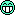# Goniometer Algorithm

DSP, Plugin and Host development discussion.
RELATED
PRODUCTS
Hawxflight
KVRer
Topic Starter
1 posts since 25 Jan, 2017
Hey,
I'm currently trying to create a goniometer - like the one in the Waves PAZ package.However I can't seem to find any algorithm that describes how to get from the samples to the angles.
I'd guess that the angles would be something like this:
-45 = Left
45 = Right
0 = Centre/mono
<45 = Out of phase
>-45 = Out of phase

I've already got an FFT component working that provides the amplitude for each sample.
Any help would be greatly appreciated!

Thanks,
HawxChris-S
KVRAF
2919 posts since 10 Nov, 2013 from Germany
Hi,
REAPER comes with a JS-script goniometer which you could check.

I think it's included in the reaplugs-package.
http://www.reaper.fm/reaplugs/

It's not FFT based (already did some local mods to the script).random_id
KVRian
503 posts since 1 May, 2006 from lancaster, pa
This page (http://dsp.stackexchange.com/questions/ ... hase-meter) has some good information about figuring out the information needed to graph.

Mayae
KVRian
564 posts since 1 Jan, 2013 from Denmark
Usually you obtain the phase of the input pair (x,y) using the arctangent. If you're plotting a standard XY scope, you'll wan't to use atan2(y, x), otherwise use atan(y / x). atan()'s output range is only a half circle as you see (-pi/2 .. pi / 2), where atan2() can produce the full circle.

The length of the line is usually just max(|x|, |y|). You may need to additionally to 2d-rotate the values -pi/2, such that an angle of pi/2 on a unit circle appears to be "in phase" as the example UI depicts.binaryoblivion
KVRist
73 posts since 18 Feb, 2016
I recently made a simple goniometer for my first Juce project. Here is some of my C++ code and comments, showing how I convert input sample data from cartesian to polar coordinates, rotate it 45 degrees, then convert back to cartesian coordinates ready to be plotted on a graph. This is my first audio plugin and C++ project so it is possible my approach is naive, but hopefully it is helpful!

Code: Select all

``````double x = points[i].second; // Right channel is mapped to x axis
double y = points[i].first; // Left channel is mapped to y axis

// Convert cartesian to polar coordinate
// @see https://www.mathsisfun.com/polar-cartesian-coordinates.html
double radius = sqrt((x * x) + (y * y));
double angle = atan(y/x);

// atan() returns wrong value if either value is negative.
// Correct for this by rotating 180 or 360 degrees depending on which
// quadrant of the x/y graph the cartesian coordinate is in.
if ((x < 0 && y > 0) || (x < 0 && y < 0)) {
angle += 3.14159265; // Pi radians = 180 degrees
} else if (x > 0 && y < 0) {
angle += 6.28318530; // 2Pi radians = 360 degrees
}

// atan() will return zero if either of our coordinates is zero.
// Correct for this by manually setting the angle.
if (x == 0) {
angle = y > 0 ? 1.57079633 : 4.71238898; // 90 or 270 degrees
} else if (y == 0) {
angle = x > 0 ? 0 : 3.14159265; // 0 or 180 degrees
}

// Rotate coordinate by 45 degrees counter clockwise
angle += 0.78539816;

// Convert polar coordinate back to cartesian coordinate.
double xRotated = radius * cos(angle);
double yRotated = radius * sin(angle);
``````
Oblivion Sound Lab
Synthesizer soundsets and audio plugins
https://oblivionsoundlab.com

CaptnWillie
KVRer
1 posts since 1 Dec, 2016
Fantastic example code - I'm starting to work on a Goniometer currently, I've already implemented a compressor with an fft using WDL-OL.

I'll have a look at your code with what i've been writing, i'm pretty new to C++ and i'm having fun figuring it out